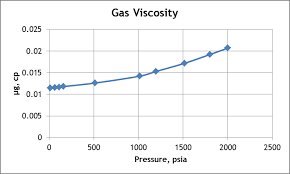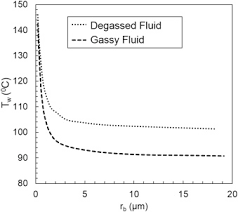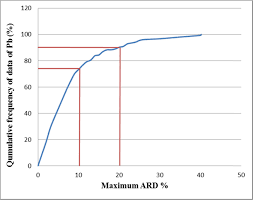## How to Calculate and Solve for MBAL Gas Solubility | The Calculator EncyclopediaThe image above represents the MBAL gas solubility.

To compute for the MBAL gas solubility, four essential parameters are needed and these parameters are Specific Gravity of Solution Gas (γg), Specific Gravity of Stock Tank Oil (γo), Oil FVF (Bo) and Oil Density (ρo).

The formula for calculating MBAL gas solubility:

Rs = Boρo – 62.4γo / 0.0136γg

Where:

Rs = MBAL Gas Solubility
γg = Specific Gravity of Solution Gas
γo = Specific Gravity of Stock Tank Oil
Bo = Oil FVF
ρo = Oil Density

Let’s solve an example;
Given that specific gravity of solution gas is 9, specific gravity of stock tank oil is 13, oil FVF is 19 and oil density is 27. Find the MBAL gas solubility?

This implies that;

γg = Specific Gravity of Solution Gas = 9
γo = Specific Gravity of Stock Tank Oil = 13
Bo = Oil FVF = 19
ρo = Oil Density = 27

Rs = Boρo – 62.4γo / 0.0136γg
Rs = 19 x 27 – 62.4 x 13 / 0.0136 x 9
Rs = 513 – 811.19 / 0.1224
Rs = -298.19 / 0.1224
Rs = -2436.27

Therefore, the MBAL gas solubility is -2436.27.

## How to Calculate and Solve for Petrosky-Farshad Gas Solubility Parameter | The Calculator EncyclopediaThe image above represents petrosky-farshad gas solubility parameter.

To compute for the petrosky-farshad gas solubility parameter, two essential parameters are needed and these parameters are API Gravity (°API) and temperature (°Rankine) (T).

The formula for calculating the petrosky-farshad gas solubility parameter:

Rs = [7.716(10-4) (°API)1.541] – [4.561(10-5) (T -460)1.3911]

where:

Rs = Petrosky-Farshad Gas Solubility Parameter, x
°API = API Gravity
T = Temperature (°Rankine)

Let’s solve an example;
Find the petrosky-farshad gas solubility parameter with an API Gravity of 11 and a temperature of 90.

This implies that;

°API = API Gravity = 11
T = Temperature (°Rankine) = 90

Rs = [7.716(10-4) (°API)1.541] – [4.561(10-5) (T -460)1.3911]
Rs = [7.716(10-4) (11)1.541] – [4.561(10-5) (90 – 460)1.3911]
Rs = [7.716(10-4) (11)1.541] – [4.561(10-5) (-370)1.3911]
Rs = [7.716(10-4) (11)1.541] – [4.561(10-5) (NaN)]
Rs = [7.716(10-4) (40.2518)] – [4.561(10-5) (NaN)]
Rs = [0.03105] – [4.561(10-5) (NaN)]
Rs = 0.03105 – NaN
Rs = NaN

Therefore, the Petrosky-Farshad gas solubility parameter, x is NaN.

## How to Calculate and Solve for Petrosky-Farshad Gas Solubility in a Fluid | The Calculator EncyclopediaThe image above represents petrosky-farshad gas solubility.

To compute for the petrosky-farshad gas solubility, three essential parameters are needed and these parameters are gas specific gravity (γg), petrosky-farshad gas solubility parameter (x) and pressure (P).

The formula for calculating the petrosky-farshad gas solubility:

Rs = [(P/112.727 + 12.340)γg0.8439 10x]1.7384

Where:

γg = Gas Specific Gravity
x = Petrosky-Farshad Gas Solubility Parameter
P = Pressure

Let’s solve an example;
Find the petrosky-farshad gas solubility when the gas specific gravity is 16, the petrosky-farshad gas solubility parameter is 22 and the pressure is 80.

This implies that;

γg = Gas Specific Gravity = 16
x = Petrosky-Farshad Gas Solubility Parameter = 22
P = Pressure = 80

Rs = [(P/112.727 + 12.340)γg0.8439 10x]1.7384
Rs = [(80/112.727 + 12.340)160.8439 x 1022]1.9384
Rs = [(0.709 + 12.340)160.8439 x 1022]1.9384
Rs = [(13.049)160.8439 x 1022]1.9384
Rs = [(13.049)10.379 x 1022]1.9384
Rs = [1.354e+24]1.9384
Rs = 8.925e+41

Therefore, the Petrosky-Farshad gas solubility is 8.925e+41.